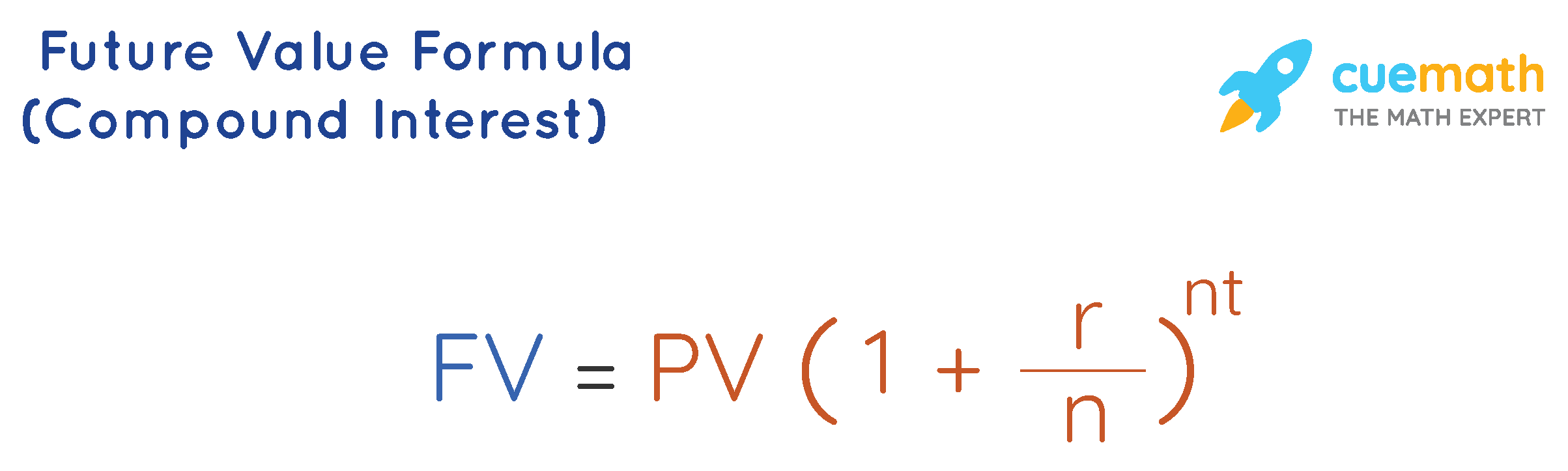# Future Value Formula Compound Interest

The word "interest" means the extra amount earned by the investor along with the investment (or) the amount owed by the borrower along with the amount lent.

Compound interest for the first period is similar to the simple interest but the difference occurs from the second period of time. From the second period, the interest is also calculated on the interest thus earned on the previous period of time, that is why it is known as interest on interest. The future value formula (compound interest) thus helps in calculating the final amount, which includes the initial investment along with total interest.

In compound interest,

• The "present value" represents the initial investment.
• The "future value" represents the final amount (initial investment + total interest).

Let us understand the future value formula(compound interest) using solved examples in the following sections.

## What is Future Value Formula (Compound Interest)?

The future value formula of compound interest is:Here,

• PV = Present Value (Initial investment)
• r = rate of interest (in decimals, divide the given percentage by 100)
• n = number of times the amount is compounding
• t = time in years

The value of n depends on the number of times the amount is compounding.

• n = 1, if the amount is compounded yearly.
• n = 2, if the amount is compounded half-yearly.
• n = 4, if the amount is compounded quart-yearly.
• n = 12, if the amount is compounded monthly.
• n = 52, if the amount is compounded weekly.
• n = 365, if the amount is compounded daily.

## Solved Examples Using Future Value Formula (Compound Interest)

### David borrowed $5000 from a bank at a rate of 7% per annum compounded annually. How much he has to pay back at the end of 4 years? Solution: To find: The future value of the borrowed amount after 4 years. The present value (investment) , PV =$5000.

The rate of interest, r = 7% =7/100 = 0.07.

The time in years, t = 4.

Since the amount is compounded annually, n = 1.

Using the future value formula of compound interest:

FV = PV (1 + r / n)n t

FV = $$5000 \left( 1+ \dfrac{0.07}{1}\right)^{1\times 4}$$

A = $6,553.98 Answer: The future value =$6,553.98.

### You have invested $1000 in a bank where your amount gets compounded daily at 5% annual interest. Then what is the future value of the amount you have invested for 10 years? Solution: To find: Fututre value for an investment after 10 years. The present value (investment), PV =$1000.

The rate of interest, r = 5% =5/100 = 0.05.

The time in years, t = 10.

Since the amount is compounded daily, n = 365.

Using the future value formula of compound interest:

FV = PV (1 + r / n)n t

FV = $$1000 \left( 1+ \dfrac{0.05}{365}\right)^{365 \times 10}$$

FV = $1648.66 Answer: The future value =$1648.66.